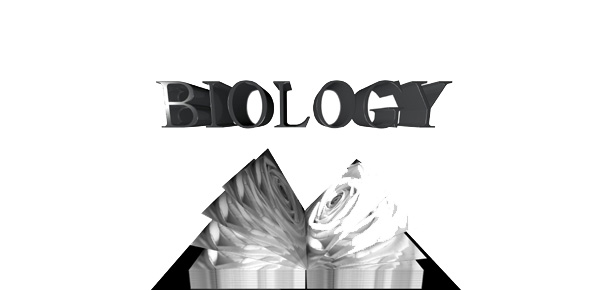# Optimality Theory

12 Questions | Total Attempts: 44SettingsQuestions from chapter 12 of Introducing Linguistics Morphology.

Related Topics
• 1.
Optimality Theory is a theory that uses rules.
• A.

True

• B.

False

• 2.
Differences in grammars reflect different _______ of the universal constraint set, CON. Part of Language acquisition can then be described as the process of adjusting the ________ of these constraints.
• 3.
Constraints are organized in a ___________ of strength, which is language specific.
• 4.
The output that is actually used (which appears on the surface) is the one which breaks the _________ constraints.
• A.

Fewest

• B.

Most

• C.

Weakest

• D.

Strongest

• 5.
A _____________ in the tableau indicates that the constraints are not ranked.
• A.

Dotted line between the two constraints

• B.

Asterisk

• C.

Solid line between the two constraints

• D.

• 6.
The ________________ indicates that nothing in the that part of the table is relevant for coming to a decision (this has no theoretical status but makes it easier to see what is going on).
• A.

Exclamation mark

• B.

• C.

Dotted line between the two constraints

• D.

Pointy hand

• 7.
The ranking of the constraints is shown by
• A.

The exclamation mark

• B.

• C.

The solid line between them in the tableau

• 8.
"We can see this type of approach (constraints) being generalized in morphological studies. It has been argued that some ______________ morphology might be best accounted for in terms of constraint interaction and the use of output constraints as a way of determining allomorphs is becoming more fashionable."
• A.

Natural

• B.

Derivational

• C.

Subtractive

• D.

Inflectional

• 9.
Optimality Theory is often called a ____________ theory of language, because it has its roots in neural network research, though the relationship is now largely of historical interest. It arose in part as a successor to the theory of Harmonic Grammar, developed in 1990 by Géraldine Legendre, Yoshiro Miyata and Paul Smolensky.
• A.

Cohort

• B.

Dual Route

• C.

Morphological Race

• D.

Connectionist

• E.

Priming

• F.

Suffixing Preference

• 10.
A language's grammar (its ranking of constraints) determines which of the infinite candidates will be assessed as optimal by _____________.
• A.

GEN

• B.

CON

• C.

EVAL

• 11.
There are two types of constraints: _________ and ___________.
• 12.
If grammars differ only by having different rankings of ________, then the set of possible human languages is determined by the constraints that exist. Optimality theory predicts that there cannot be more grammars than there are permutations of the ranking of _______.
• A.

GEN

• B.

CON

• C.

EVAL